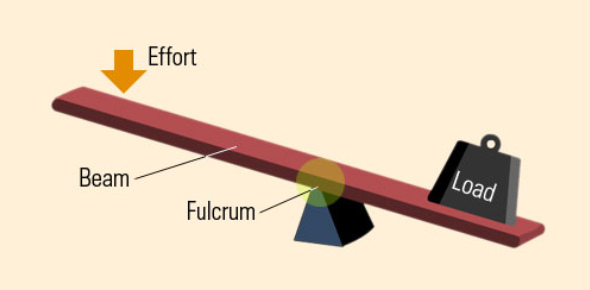# Quiz On Levers And Simple Machines MCQ With Answers

13 Questions | Total Attempts: 14044SettingsHow much do you know about levers from our classes? Take this quiz on levers and simple machines MCQ with answers to learn about simple machines. Most of the machines we use today have a lever or two, decreasing the force it would take to get a job done. A lever is a rigid body capable of rotating on a point itself. Play the quiz below and see if you understood all we covered on the topic and the application in real life.

• 1.
A benefit obtained by using a lever is called a(n)
• A.

Newton

• B.

• C.

Interval

• D.

Effort

• 2.
As the load is moved farther from the fulcrum, the effort needed to lift the load.
• A.

Increases

• B.

Decreases

• C.

Stays the same

• D.

Is the opposite

• 3.
The unit used to measure the amount of force (effort) needed to lift a load is the:
• A.

Kilogram

• B.

Lever arm

• C.

Class 2 lever

• D.

Newton

• 4.
What kind of lever system is a hockey stick?
• A.

Class 1

• B.

Class 2

• C.

Class 3

• D.

A hockey stick is not a lever.

• 5.
A crowbar is a class _____ lever.
• A.

1

• B.

2

• C.

3

• D.

4

• 6.
What is the relationship between the amount of effort required and its distance from the fulcrum?
• A.

The farther from the fulcrum the effort is, the more effort needed.

• B.

The farther from the fulcrum the effort is, the less effort needed.

• C.

The farther from the fulcrum the effort is, the equal effort needed.

• D.

All of the above

• 7.
A class 3 lever has the _________ in the middle.
• A.

Effort

• B.

• C.

Fulcrum

• D.

All of these

• 8.
A class 2 lever has the _____ in the middle.
• A.

Effort

• B.

• C.

Fulcrum

• D.

All of these

• 9.
A class 1 lever has the _____ in the middle.
• A.

Effort

• B.

• C.

Fulcrum

• D.

All of these

• 10.
In ____________ levers, the effort increases as the load move closer to the fulcrum.
• A.

Class 1

• B.

Class 2

• C.

Class 3

• D.

Class 4

• 11.
In a __________ Lever, the Force is between the Load and the Fulcrum.
• A.

Class Three

• B.

Class Four

• C.

Class Two

• D.

Class One

• 12.
The human arm is a
• A.

Class 2 lever

• B.

Class 3 lever

• C.

Class 4 lever

• D.

None of the above

• 13.
The farther from the fulcrum the effort is, the __________ is required to lift the load.
• A.

Less effort

• B.

More effort

• C.

Equal effort

• D.

None of the above

Related TopicsBack to top